Author: Oscar Cronquist Article last updated on June 16, 2018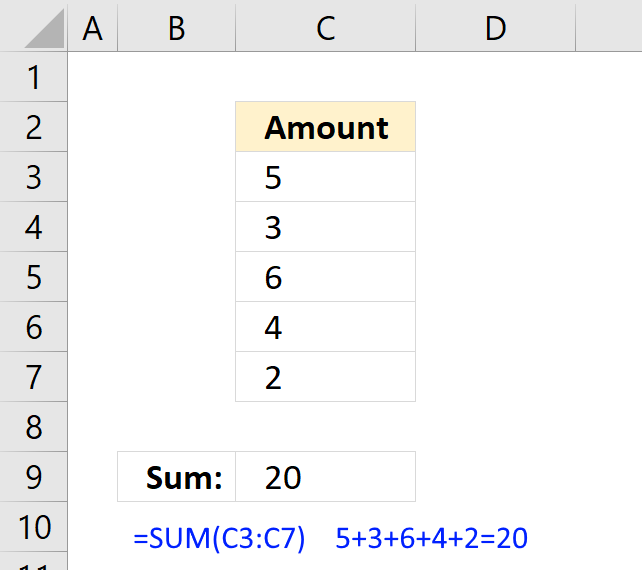The SUM function in Excel allows you to add values, the function returns the sum in the cell it is entered in. The SUM function is cleverly designed to ignore text and boolean values, adding only numbers.

### Excel Function Syntax

SUM(number1, [number2], ...)

#### Arguments

 number1 Required. A constant, cell reference or an array that contains numerical values you want to add. [number2] Optional. Up to 254 additional arguments.

The SUM function lets you add values in cell ranges, arrays, constants. You can have up to 255 different arguments.

### Add values in a cell range

The SUM function lets you add values in a cell range, like this = SUM(B3:B7), instead of adding values in a formula using the plus sign, like this =B3+B4+B5+B6+B7.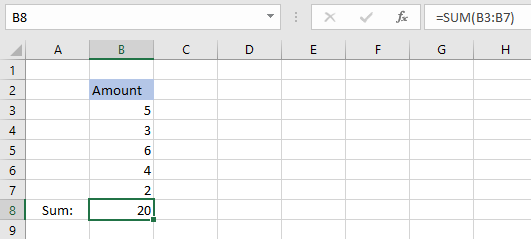The SUM function lets you type one or multiple cell ranges, in this example only cell range B3:B7 is entered as an argument. See above picture.

### Add values in an array

An array is multiple values enclosed with a beginning and ending curly bracket, you can easily convert a cell range to an array. See instructions below.

Select a cell and type =SUM(B3:B9)Click in the formula bar and select B3:B9.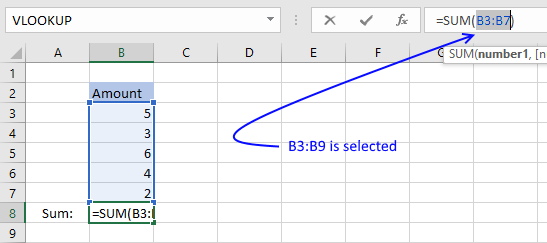Press F9 and the cell range is converted to an array, like this: =SUM({5,3,6,4,2})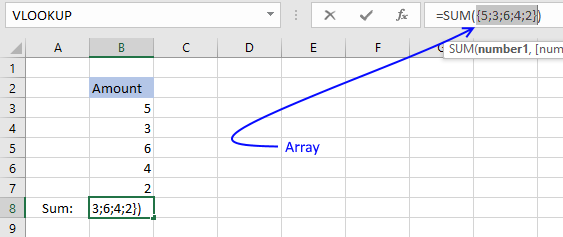Press Enter.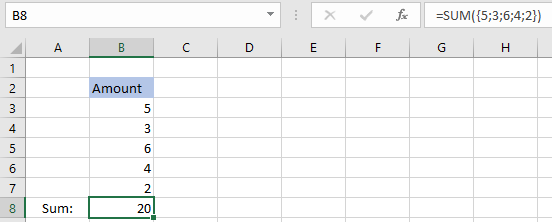The SUM function adds the values in the array 5+3+6+4+2 = 20. When you convert a cell range to values you hard-code or create constants in your formula, meaning they never change unless you change the values in the formula.

Cell references on the other hand change if you change the values on a worksheet.

### Add values in multiple cell ranges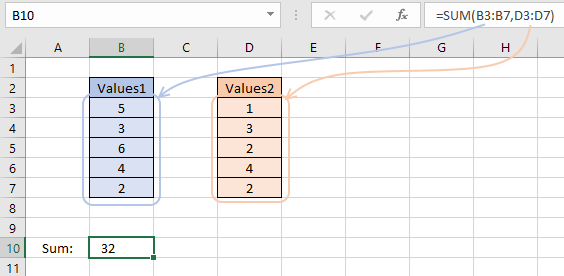If you want to add values in multiple cell ranges you simply use a comma between arguments. Check your regional settings if a comma doesn't work for you. You are allowed to have up to 255 arguments in one SUM function.

### Add numbers and ignore text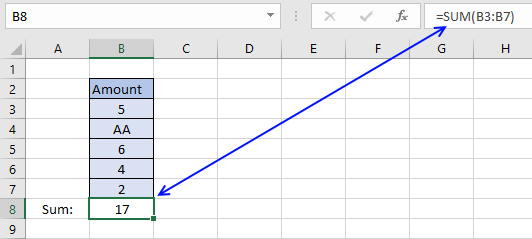The formula in cell B8 adds the values in cell range B3:B7. 5 + AA + 6 + 4 +2 = 17. The SUM function ignores text strings, in this case AA.

Cell range B3:B7 contains boolean values, TRUE or FALSE, however SUM can't add boolean values. Don't worry, there is a work-around.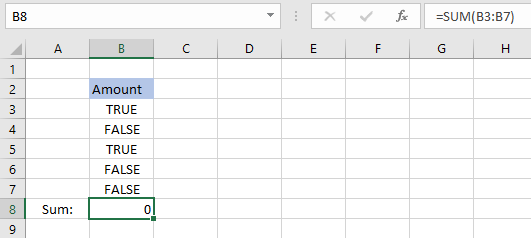There are multiple solutions to this problem, here are a few:

=SUM(--(B3:B7))

=SUM(B3:B7+0)

=SUM(B3:B7*1)

They need to be entered as array formula, because they do calculations to a cell range containing multiple cells. Instructions below on how to enter an array formula.

1. Double click cell B8
2. Type =SUM(B3:B7*1)
3. Press CTRL + SHIFT simultaneously
4. Press Enter once
5. Release all keys

The formula in the formula bar changes to {=SUM(B3:B7*1)}

These curly brackets tells you that you have created an array formula, don't enter these characters yourself.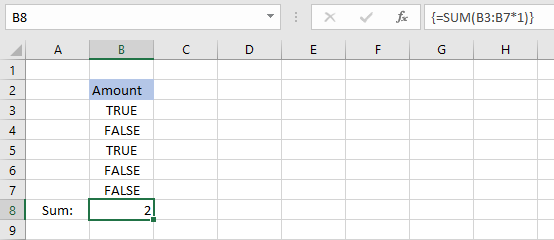The formula returns 2 because TRUE equals 1 and FALSE equals 0. 1+0+1+0+0 = 2.

### Sum using an expanding cell reference

The following picture shows you numbers in column B.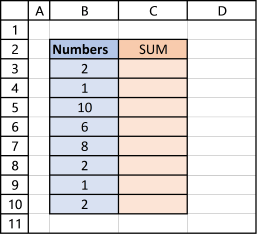Enter this formula in cell C3:

=SUM(\$B\$3:B3)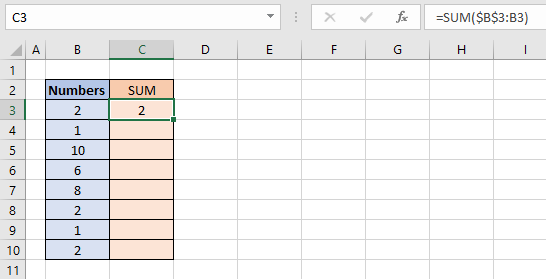Make sure you get the dollar signs right, they are important. The cell reference changes as you copy the formula and paste it to cells below.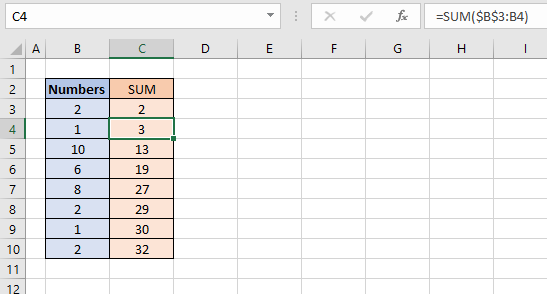Select cell C4 and see how the formula changed in the formula bar. The part of the cell reference without dollar signs changed from B3 to B4.

That part is a relative cell reference and the part with dollar signs is an absolute cell reference.
Read more here: Absolute and relative cell references

### Sum with a condition [array formula]

The picture below shows you two columns. Column B contains text values and column C contains numbers.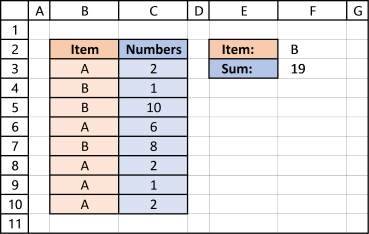The formula in cell F3 lets you add numbers in column C if their adjacent value is equal to the value in cell F2:

=SUM((B3:B10=F2)*C3:C10)

This formula is an array formula, read Boolean values above how to enter an array formula.

The equal sign in B3:B10=F2 lets you compare the values in cell range B3:B10 with the value in cell F2. The equal sign is a logical operator, often used in IF functions.

This logical test returns an array of boolean values, in this case {FALSE; TRUE; TRUE; FALSE; TRUE; FALSE; FALSE; FALSE} The parentheses (B3:B10=F2) makes sure this part of the formula is calculated first before multiplying with the numbers in cell range C3:C10.

(B3:B10=F2)*C3:C10 becomes {FALSE; TRUE; TRUE; FALSE; TRUE; FALSE; FALSE; FALSE}*C3:C10 and returns {0; 1; 10; 0; 8; 0; 0; 0}

The SUM function then adds the number in the array: SUM({0; 1; 10; 0; 8; 0; 0; 0}) and returns 19 in cell F3. 1+10+8 = 19

Tip! The powerful excel table can do all this for you:
Excel defined table - SUM with criteria

### Sum with multiple conditions [array formula]

Adding a second condition to the formula is easy. Simply add your condition to the formula enclosed with parentheses.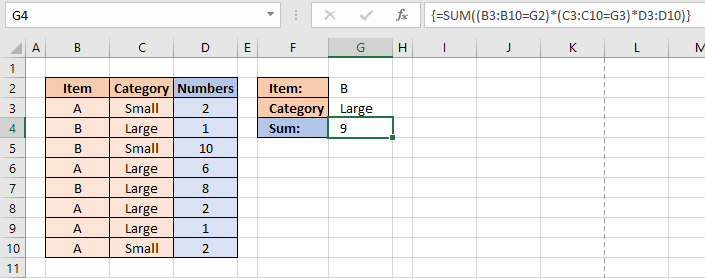=SUM((B3:B10=G2)*(C3:C10=G3)*D3:D10)

### SUM - VBA example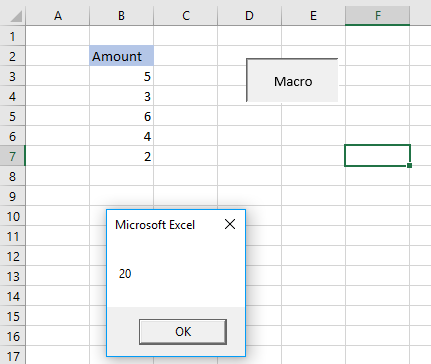```Sub HLP()
MsgBox Application.WorksheetFunction.Sum(Range("B3:B7"))
End Sub
```